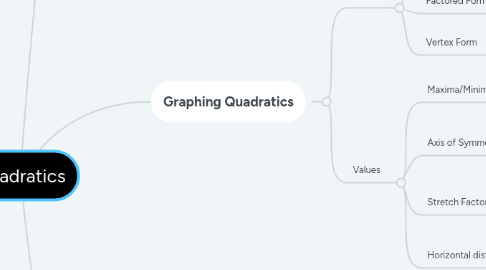Nirojan Angusamoorthy
Get Started. It's FreeQuadratics## 1. Factoring

### 1.1. Common Factoring

1.1.1. Factoring Trinominals (3 Terms)

1.1.1.1. Complex Trinominal (when coefficient is a>1

1.1.1.1.1. ax^2 + bx + c

1.1.1.2. Simple Trinominal (when coefficient is a=1)

1.1.1.2.1. x^2 + bx + c

1.1.1.3. Perfect Square Trinominal

1.1.1.3.1. a^2 + 2ab + b^2 = (a +b)^2

1.1.1.3.2. a^2 - 2ab + b^2 = (a-b)^2

1.1.2. Factoring Binominals (2 Terms)

1.1.2.1. Difference of Squares

1.1.2.1.1. a^2 - b^2 = (a+b)(a-b)

1.1.2.2. Common Factoring Binominals

1.1.2.2.1. a(x-y)-b(x-y)

1.1.3. Factoring By Grouping (4 terms)

1.1.3.1. Find the GCF between 2 pairs.

1.1.3.1.1. Ex. x^3 - 6x^2 + 10x - 60 ---> =x^2[x-6]+10[x-6]

1.2.1. Completing the Square

1.2.1.1. Convert Standard form to Vertex form to solve it

1.2.1.1.1. Factor all terms by the a (ex. 2x^2 + 4x + 4 -----> 2(x^2 + 2x + 2)

1.2.2. Quadratic Formula (Only used in Standard Form)

1.2.2.1. Solving Quadratics Equations from Standard form

1.2.3. Discriminant Formula

1.2.3.1. Reveals what type of roots the equation would have

1.2.3.1.1. Root>0

1.2.3.1.2. Root=0

1.2.3.1.3. Root<0

## 2. Expanding

### 2.1. Expanding different types

2.1.1. Expanding Binominals

2.1.1.1. Method (F)irst (O)utside (I)nside (L)

2.1.1.1.1. (a+b)(c+d) = ac + ad + bc + bd

2.1.2. Product of the Sum and Difference (no middle term)(similar to difference of squares in factoring)

2.1.2.1. (a+b)(a-b) = a^2 - b^2

2.1.3. Perfect Square Trinomials (similar to factoring perfect square trinominals but expanding)

2.1.3.1. (a+b)^2 = a^2 + 2ab + b^2

2.1.3.2. (a-b)^2 = a^2 -2ab + b^2

## 3. Common Misconceptions

### 4.1. Forms

4.1.1. Standard Form

4.1.1.1. y=ax^2 + bx + c

4.1.1.1.1. The standard form helps you find the y - intercept and can help you find the x value (quadratic formula)

4.1.2. Factored Form

4.1.2.1. y=a(x-r)(x-s)

4.1.2.1.1. This form can help you find the x-intercepts in a graph (the zeroes)

4.1.3. Vertex Form

4.1.3.1. y=a(x-h)^2 + k

4.1.3.1.1. This form can help you find the vertex in the graph (k value)(x value)

### 4.2. Values

4.2.1. Maxima/Minima

4.2.1.1. The maxima/minima are either the highest point in the graph, or lowest point of the graph

4.2.1.1.1. You can find these values in vertex form

4.2.2. Axis of Symmetry (x value in vertex form)

4.2.2.1. The axis of symmetry is in the center of the 2 x - ints

4.2.2.1.1. You can find these values either in the vertex form or factored form (average the zeroes)

4.2.3. Stretch Factor (a value)

4.2.3.1. The stretch factor determines if the graph is reflected and how wide/thin it is

4.2.3.1.1. You can use the a value to get a step pattern by multiplying 1 to get the first point, and multiplying by 2 to get the same point

4.2.4. Horizontal distance (h value)

4.2.4.1. The horizontal distance determines where the graph starts on the x axis

4.2.4.1.1. You can find this value in the vertex form (the h value)